Next: 6.4 Low level relational Up: 6 Creating New Relations Previous: 6.2 Binary relational operations

# 6.3 Unary relational operations

Relation Transitive_Closure(Relation &r, Relation & IterationSpace=Relation::Null())
Works for relations only. The input and output arity of r and arity of IterationSpace should be the same. If r is a dependence relation, IterationSpace is a set describing iteration space of r. Parameter IterationSpace can be omitted. In this case the techniques requiring information about it are not employed.
Computing transitive closure exactly for all relations is undecidable, sinceencodes multiplication; Presburger plus multiplication is undecidable.
In cases where we cannot compute the exact transitive closure, we compute a lower bound. Details can be found elsewhere [KPRS95].

Relation Domain(Relation &r)
Works for relations only. Ifthen the result isRelation Range(Relation &r)
Works for relations only. Ifthen the result isRelation Inverse(Relation &r)
Works for relations only. Ifthen the result isRelation Complement(Relation &r)
Works for both relations and sets. Ifthen the result isRelation Hull(Relation &r, bool stridesAllowed = false)
Works for both relations and sets. We guaranteed thatand thatis described by a single conjunct. If strides are allowed, the result may contain stride constraints (e.g., i is even). Other than this, no guarantees are made. The Hull function might simply return True, which satisfies all of the stated conditions. We try to do better than this, but no promises. The result is not necessarily ``the convex hull'' of r
Relation Identity(int n)
The argument must be non-negative. The result is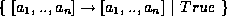Relation Project(Relation &r, Global_Var_ID v)
Works with both relations and sets. Ifthen the result is, where f is the same as fexcept that all occurrences of variable v are replaced by variable z.
Relation Project(Relation &r, int pos, Var_Type vtype)
Works with both relations and sets. If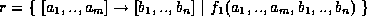then the result is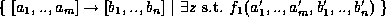, whereexcept thatif vtype is Input_Var andif vtype = Output_Var.
Relation Project_Sym(Relation &r)
Works with both relations and sets. If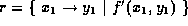then the result is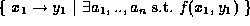, where f is the same as fexcept that all occurrences of each global variableare replaced by variable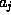.
Relation Project_On_Sym(Relation &r)
Works with both relations and sets. Ifthen the result is.
Relation Extend_Domain(Relation &r)
Works with relations only. Ifthen the result is,
Relation Extend_Domain(Relation &r, int n)
Equivalent of applying the simple Extend_Domain function n times.
Relation Extend_Range(Relation &r)
Works with relations only. Ifthen the result is,
Relation Extend_Range(Relation &r, int n)
Equivalent of applying the simple Extend_Range function n times.
Relation Extend_Set(Relation &r)
Works with sets only. If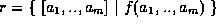then the result is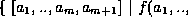,
Relation Extend_Set(Relation &r, int n)
Equivalent of applying the simple Extend_Set function n times.
Relation Deltas(Relation &r)
Works for relations only. The input arity must be the same as the output arity. If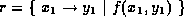then the result isRelation Deltas(Relation &r, int p)
Works with relations only. Ifthen it must be the case thatand the result is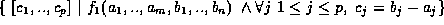Relation Approximate(Relation &r)
Works for both relations and sets. Ifthen the result is, where f is the same as fexcept that all quantified variables are henceforth designated as being able to have non-integer rational values. The effect of this designation is that all of these variables will be able to eliminated exactly (via Fourier variable elimination) when the formula is simplified.
Relation Approximate(Relation &r, int strides_allowed)
Works for both relations and sets. If strides_allowed is False then the result is the same as the simple Approximate function. If strides_allowed is True then the result is the same as the simple Approximate function except that if a quantified variable is only involved in one constraint then it is not designated as being able to have non-integer rational values. The effect of this function is that the only variables that can't be eliminated exactly, are those that correspond to simple stride constraints.
Relation EQs_to_GEQs(Relation &r, bool excludeStrides=false)
Works for both relations and sets. Ifthen the result is, where fis the same as f except that all equality constraints are converted into a matching pair of inequalities. If excludeStrides is True then this convertion process is not applied to those equality constraints that correspond to stride constraints (i.e. those constraints involving a single quantified variable).
Relation Sample_Solution(Relation &r)
For a relation R, returns a relation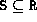where each variable in S has exactly one value.
Relation Symbolic_Solution(Relation &r)
For a relation R, returns a relationwhere each input, output, or set variable in S has exactly one value, plus constraints on the symbolic variables.Next: 6.4 Low level relational Up: 6 Creating New Relations Previous: 6.2 Binary relational operations

omega@cs.umd.edu

Web Accessibility